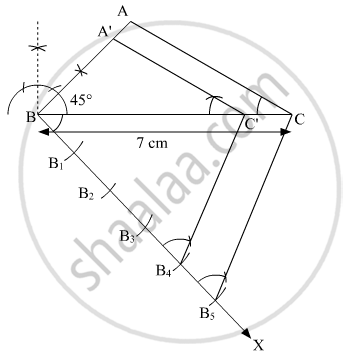# Draw a triangle ABC with BC = 7 cm, ∠B = 45° and ∠A = 105°. Then construct a triangle whose sides are 4/5 times the corresponding sides of ΔABC. - Mathematics

Sum

Draw a triangle ABC with BC = 7 cm, ∠B = 45° and ∠A = 105°. Then construct a triangle whose sides are4/5 times the corresponding sides of ΔABC.

#### Solution

Given:
∠B = 45°
∠A = 105°

Sum of all interior angles in a triangle is 180°.
∠A + ∠B + ∠C = 180°

105° + 45° + ∠C = 180°

∠C = 180° − 150°

∠C = 30°

Following steps are involved in the construction of the required triangle:

Step 1

Draw a ΔABC with side BC = 7 cm, ∠B = 45° and ∠C = 30°.

Step 2

Draw a ray BX making an acute angle with BC on the opposite side of vertex A.

Step 3

Locate 5 points (as 5 is greater in 5 and 4) B1, B2, B3, B4 and Bon BX such that BB1, B1B2, B2B3, B3B4 and B4B5.

Step 4

Join B5C. Draw a line through B4 parallel to B5C intersecting extended BC at C'.

Step 5

Through C', draw a line parallel to AC intersecting BA at A'.

ΔA'BC' is the required triangle.Justification

The construction can be justified by proving that

A'B=4/5AB,BC'=4/5BC andA'C,= 4/5AC

In ΔABC and ΔA'BC',

∠ABC = ∠A'BC' (Common)

∠ACB = ∠A'C'B (Corresponding angles)

∴ ΔABC ∼ ΔA'BC' (AA similarity criterion)

(AB)/(A'B)=(BC)/(BC')=(AC)/(A'C')

In ΔBB5C and ΔBB4C',

∠B4BC' = ∠B5BC (Common)

∠BB4C' = ∠BB5C' (Corresponding angles)

∴ ΔBB4C' ∼ ΔBB5C (AA similarity criterion)

(BC')/(BC)=(BB_4)/(BB_6)

=>(BC')/(BC)=4/5

Comparing (1) and (2), we obtain

(A'B)/(AB)=(BC')/(BC)=(A'C')/(AC)=4/5

This justifies the construction.

Concept: Division of a Line Segment
Is there an error in this question or solution?
Chapter 11: Constructions - Exercise 11.1 [Page 220]

#### APPEARS IN

NCERT Class 10 Maths
Chapter 11 Constructions
Exercise 11.1 | Q 6 | Page 220

Share